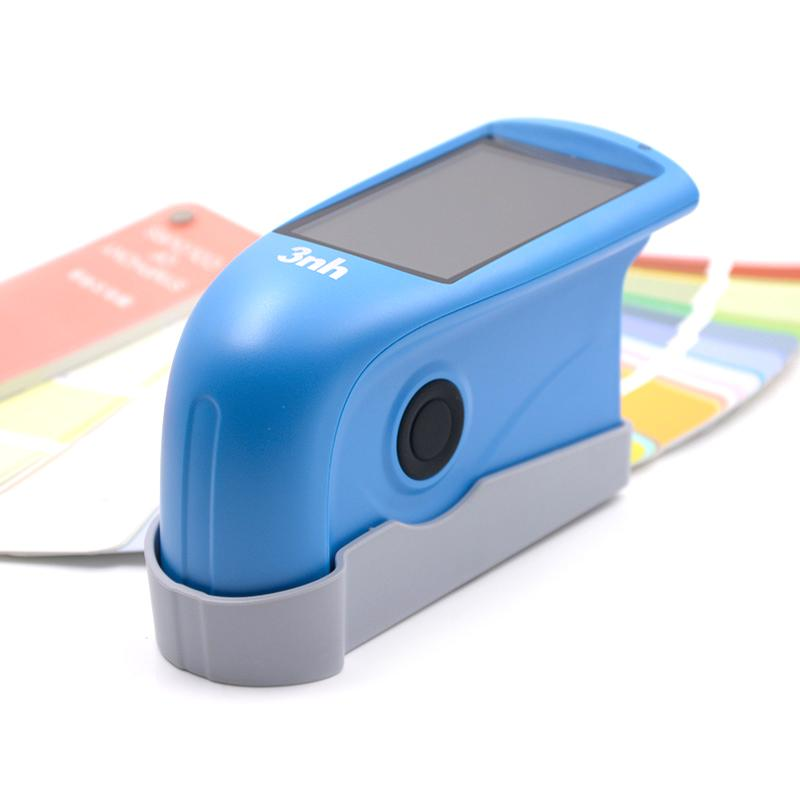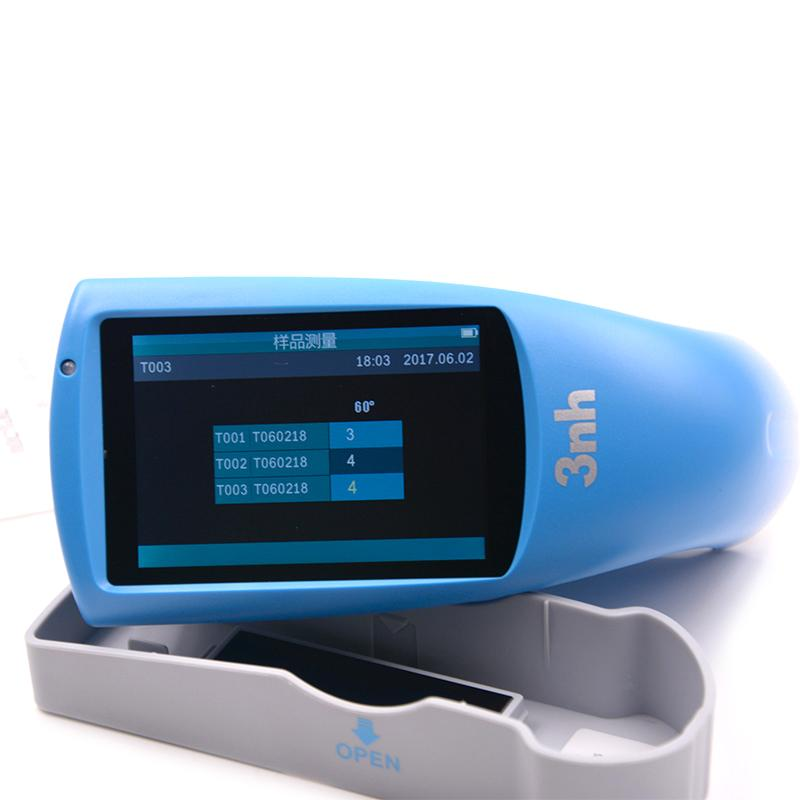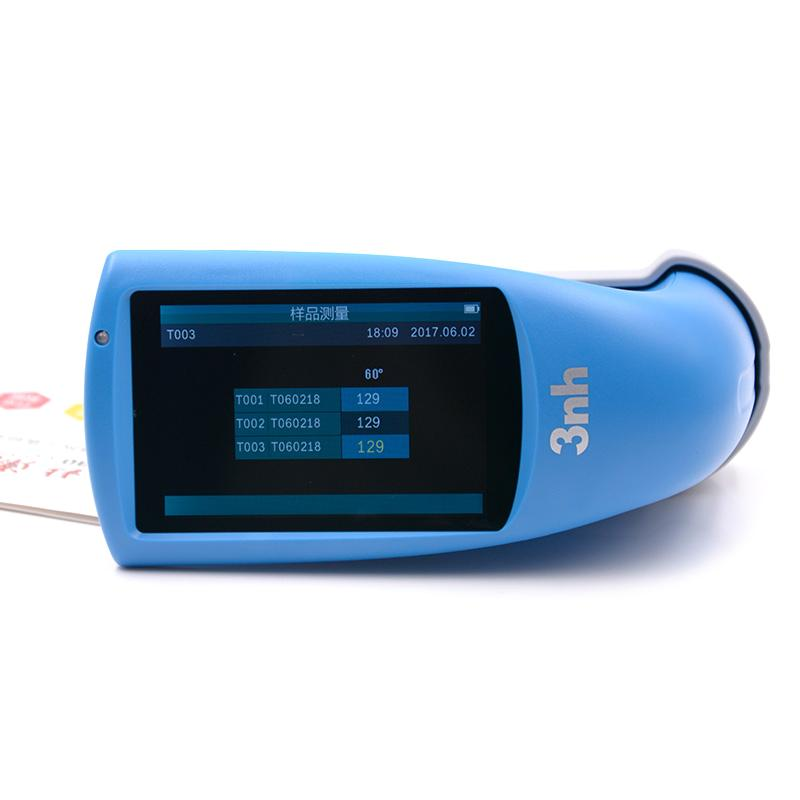# Gloss unit GU and % how to calculate

Glossometer is usually used to measure the glossiness of the surface of the object. Common glossiness units are GU and %.GU stands for GLoss Units. Gu represents the number in the glossometer’s measurements, and % represents the relative glossiness percentage of the number compared to the standard sample.

Gloss unit GU and % how to calculate

The specific calculation method is as follows:

For GU, the glossometer gives a number by comparing the difference between the Angle of reflection from a standard light source and the Angle of reflection from the test sample.This number is the GU value, which usually ranges from 0 to 200.

For %, the standard sample is defined as 100GU.Therefore, GU can be converted to relative gloss percentage by the following formula: Relative gloss %= (sample GU value Standard sample GU value) X100% For example, if the GU value of the standard sample is 100 and the GU value of the test sample is 80, then the relative gloss is 80÷100 X100% = 80%.

It is important to remind you that different glossometers may use different standard light sources and geometric reflection angles, so the results may be different when measured on different devices.